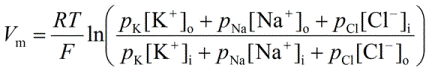PhysiologyWeb  Home  |  FAQ  |  About  |  Contact  |   - Sign InTweetPhysiology Quiz -
The Membrane Potential - Part 3
Cells of multicellular organisms such as animals and plants, as well as those of unicellular organisms such as yeast exhibit a potential difference across the cell plasma membrane. That is to say that there is an electrical potential difference between the inside of the cell and the surrounding bathing medium of the cell. This potential difference is referred to as the membrane potential (Vm). The numerical value of the membrane potential is generally negative, meaning that the inside of the cell is negative with respect to the outside solution, which is taken as the reference or zero value.

Test your knowledge of the membrane potential by taking this series of quizzes. For more information about the membrane potential, see the Resting Membrane Potential lecture notes.
(1)   A change in the membrane potential (Vm) from the resting value of −50 mV to a new value of −90 mV is referred to as:
 (A)   Depolarization (B)   Hyperpolarization
(2)   A change in the membrane potential (Vm) from the resting value of −50 mV to a new value of −10 mV is referred to as:
 (A)   Depolarization (B)   Hyperpolarization
(3)   In a typical cell in which K+, Na+, and Cl contribute to the value of the resting membrane potential (Vm), the following equation can be used to calculate the value of Vm.
 (A)   The Nernst equation(B)   The Goldman-Hodgkin-Katz (GHK) equation(4)   Assume that in a neuron, the plasma membrane permeability values for potassium (K+), sodium (Na+), and Cl are the following: pK = 1, pNa = 12, and pCl = 0.5.

Based on physiological concentrations of K+, Na+, and Cl (see here), determine the membrane potential in this neuron.
 (A)   −40 mV (B)   +40 mV (C)   0 mV (D)   −70 mV (E)   −90 mVPosted: Wednesday, August 13, 2014
Last updated: Monday, August 11, 2014